# Neural network implemetation - classification

This second part will cover the logistic classification model and how to train it.

While the previous section described a very simple one-input-one-output linear regression model, this tutorial will describe a binary classification neural network with two input dimensions. This model is known in statistics as the logistic regression model. This network can be represented graphically as: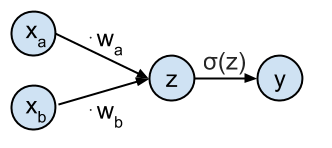In :

## Define the class distributions ¶

In this example the target classes $t$ will be generated from 2 class distributions: blue circles ($t=1$) and red stars ($t=0$). Samples from both classes are sampled from their respective distributions. These samples are plotted in the figure below. Note that $\mathbf{t}$ is a $N \times 1$ vector of target values $t_i$, and $X$ is a corresponding $N \times 2$ matrix of individual input samples $[x_{ai}, x_{bi}]$. In what follows we will also sometimes refer to the $i$-th sample of $X$ as $\mathbf{x}_i$ which is a vector of size $2$.

In :
In :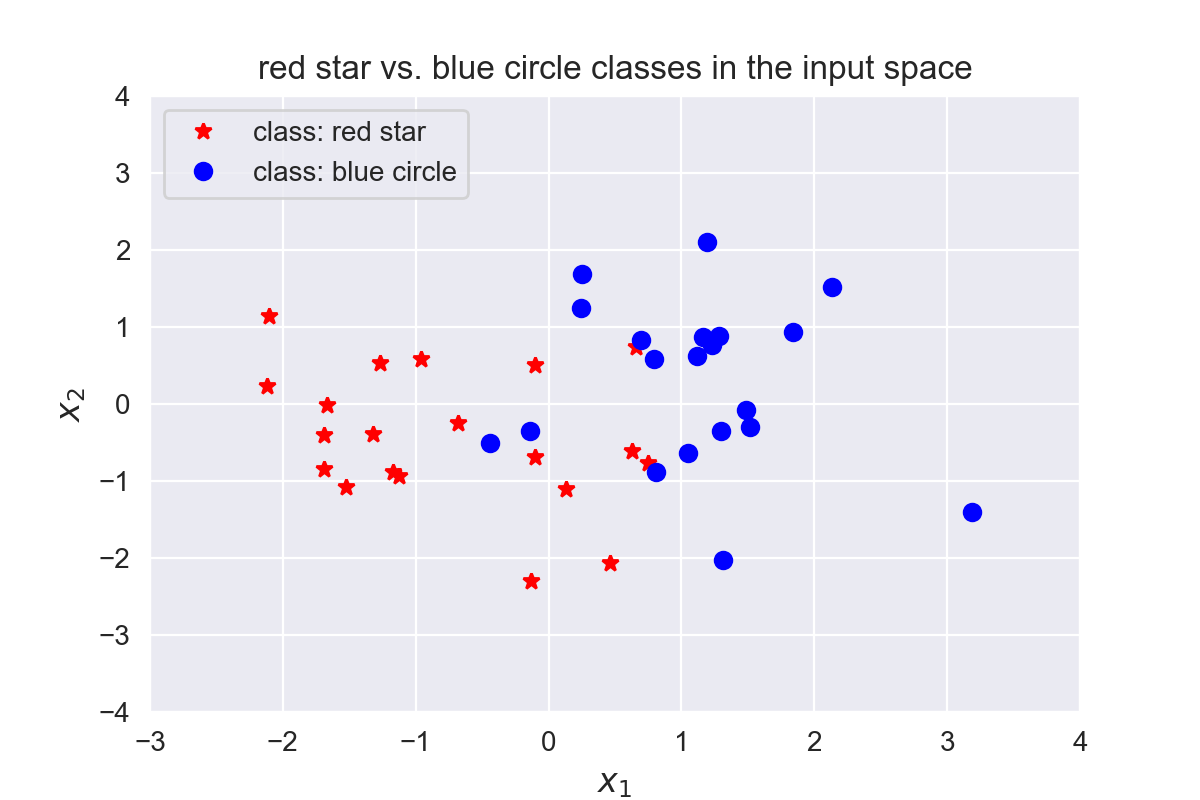## Logistic function and cross-entropy loss function ¶

### Logistic function ¶

The goal is to predict the target class $t_i$ from the input values $\mathbf{x}_i$. The network is defined as having an input $\mathbf{x}_i = [x_{ai}, x_{bi}]$ which gets transformed by the weights $\mathbf{w} = [w_a, w_b]$ to generate the probability that sample $\mathbf{x}_i$ belongs to class $t_i = 1$. This probability $P(t_i=1| \mathbf{x}_i,\mathbf{w})$ is represented by the output $y_i$ of the network computed as $y_i = \sigma(\mathbf{x}_i \cdot \mathbf{w}^T)$. $\sigma$ is the logistic function and is defined as: $$\sigma(z) = \frac{1}{1+e^{-z}}$$

The logistic function is implemented below by the  logistic(z)  method below.

### Cross-entropy loss function ¶

The loss function used to optimize the classification is the cross-entropy error function . And is defined for sample $i$ as:

$$\xi(t_i,y_i) = -t_i log(y_i) - (1-t_i)log(1-y_i)$$

Which will give $\xi(t,y) = - \frac{1}{N} \sum_{i=1}^{n} \left[ t_i log(y_i) + (1-t_i)log(1-y_i) \right]$ if we average over all $N$ samples.

The loss function is implemented below by the  loss(y, t)  method, and its output with respect to the parameters $\mathbf{w}$ over all samples $X$ is plotted in the figure below.

The neural network output is implemented by the  nn(x, w)  method, and the neural network prediction by the  nn_predict(x,w)  method.

The logistic function with the cross-entropy loss function and the derivatives are explained in detail in the tutorial on the logistic classification with cross-entropy .

In :
# Define the logistic function
def logistic(z):
return 1. / (1 + np.exp(-z))

# Define the neural network function y = 1 / (1 + numpy.exp(-x*w))
def nn(x, w):
return logistic(x.dot(w.T))

# Define the neural network prediction function that only returns
#  1 or 0 depending on the predicted class
def nn_predict(x,w):
return np.around(nn(x,w))

# Define the loss function
def loss(y, t):
return - np.mean(
np.multiply(t, np.log(y)) + np.multiply((1-t), np.log(1-y)))

In :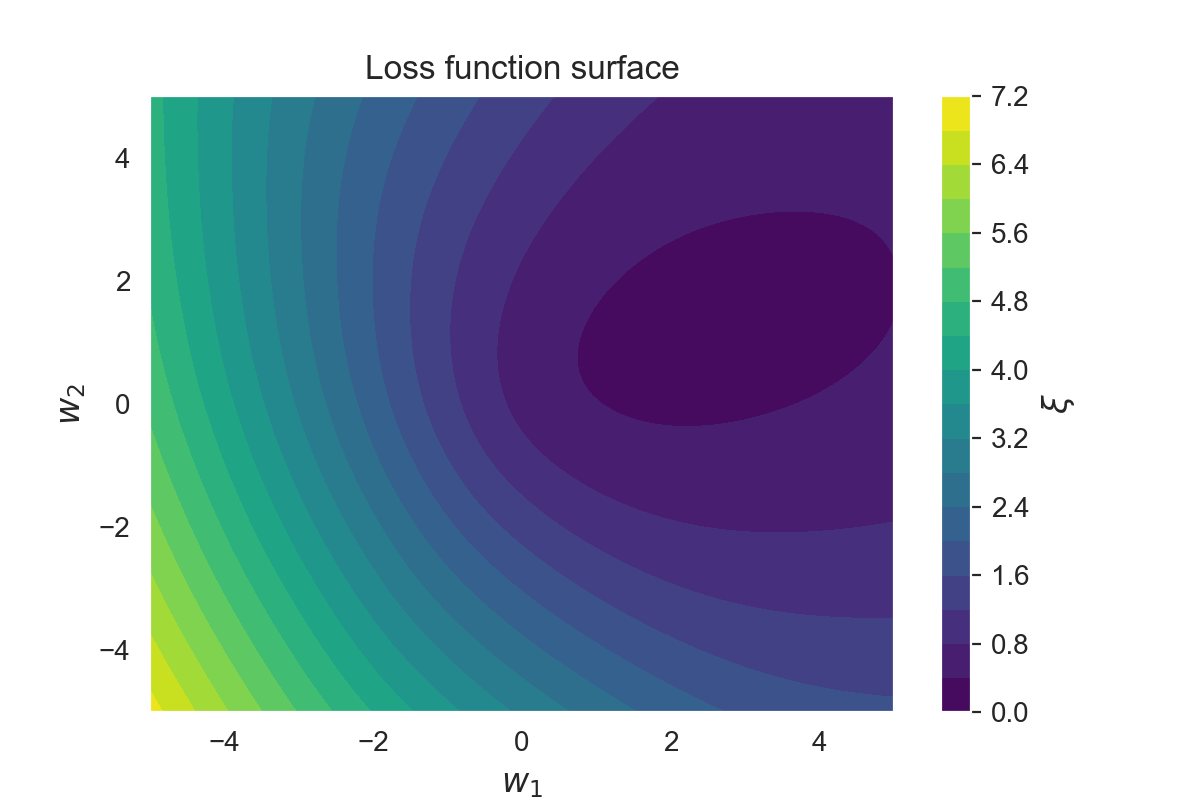## Gradient descent optimization of the loss function ¶

The gradient descent algorithm works by taking the gradient ( derivative ) of the loss function $\xi$ with respect to the parameters $\mathbf{w}$, and updates the parameters in the direction of the negative gradient (down along the loss function).

The parameters $\mathbf{w}$ are updated every iteration $k$ by taking steps proportional to the negative of the gradient: $\mathbf{w}(k+1) = \mathbf{w}(k) - \Delta \mathbf{w}(k+1)$. $\Delta \mathbf{w}$ is defined as: $\Delta \mathbf{w} = \mu \frac{\partial \xi}{\partial \mathbf{w}}$ with $\mu$ the learning rate.

Following the chain rule then ${\partial \xi_i}/{\partial \mathbf{w}}$, for each sample $i$ can be computed as follows:

$$\frac{\partial \xi_i}{\partial \mathbf{w}} = \frac{\partial \xi_i}{\partial y_i} \frac{\partial y_i}{\partial z_i} \frac{\partial z_i}{\partial \mathbf{w}}$$

Where $y_i = \sigma(z_i)$ is the output of the logistic neuron, and $z_i = \mathbf{x}_i \cdot \mathbf{w}^T$ the input to the logistic neuron.

• ${\partial \xi_i}/{\partial y_i}$ can be calculated as (see this post for the derivation):

$$\frac{\partial \xi_i}{\partial y_i} = \frac{y_i - t_i}{y_i (1 - y_i)}$$

• ${\partial y_i}/{\partial z_i}$ can be calculated as (see this post for the derivation):

$$\frac{\partial y_i}{\partial z_i} = y_i (1 - y_i)$$

• ${\partial z_i}/{\partial \mathbf{w}}$ can be calculated as:

$$\frac{\partial z_i}{\partial \mathbf{w}} = \frac{\partial (\mathbf{x}_i \cdot \mathbf{w})}{\partial \mathbf{w}} = \mathbf{x}_i$$

Bringing this together we can write:

$$\frac{\partial \xi_i}{\partial \mathbf{w}} = \frac{\partial \xi_i}{\partial y_i} \frac{\partial y_i}{\partial z_i} \frac{\partial z_i}{\partial \mathbf{w}} = \mathbf{x}_i \cdot y_i (1 - y_i) \cdot \frac{y_i - t_i}{y_i (1-y_i)} = \mathbf{x}_i \cdot (y_i-t_i)$$

Notice how this gradient is the same (negating the constant factor) as the gradient of the squared error regression from previous section.

So the full update function $\Delta \mathbf{w}$ for the weights will become:

$$\Delta \mathbf{w} = \mu \cdot \frac{\partial \xi_i}{\partial \mathbf{w}} = \mu \cdot \mathbf{x}_i \cdot (y_i - t_i)$$

In the batch processing, we just average all the gradients for each sample:

$$\Delta \mathbf{w} = \mu \cdot \frac{1}{N} \sum_{i=1}^{N} \mathbf{x}_i (y_i - t_i)$$

To start out the gradient descent algorithm, you typically start with picking the initial parameters at random and start updating these parameters according to the delta rule with $\Delta \mathbf{w}$ until convergence.

The gradient ${\partial \xi}/{\partial \mathbf{w}}$ is implemented by the  gradient(w, x, t)  function. $\Delta \mathbf{w}$ is computed by the  delta_w(w_k, x, t, learning_rate)  .

In :
def gradient(w, x, t):
return (nn(x, w) - t).T * x

def delta_w(w_k, x, t, learning_rate):
"""Update function which returns the update for each
weight {w_a, w_b} in a vector."""
return learning_rate * gradient(w_k, x, t)


Gradient descent is run on the example inputs $X$ and targets $\mathbf{t}$ for 10 iterations. The first 3 iterations are shown in the figure below. The blue dots represent the weight parameter values $\mathbf{w}(k)$ at iteration $k$.

In :
# Set the initial weight parameter
w = np.asmatrix([-4, -2])  # Randomly decided
# Set the learning rate
learning_rate = 0.05

w_iter = [w]  # List to store the weight values over the iterations
for i in range(nb_of_iterations):
dw = delta_w(w, X, t, learning_rate)  # Get the delta w update
w = w - dw  # Update the weights
w_iter.append(w)  # Store the weights for plotting

In :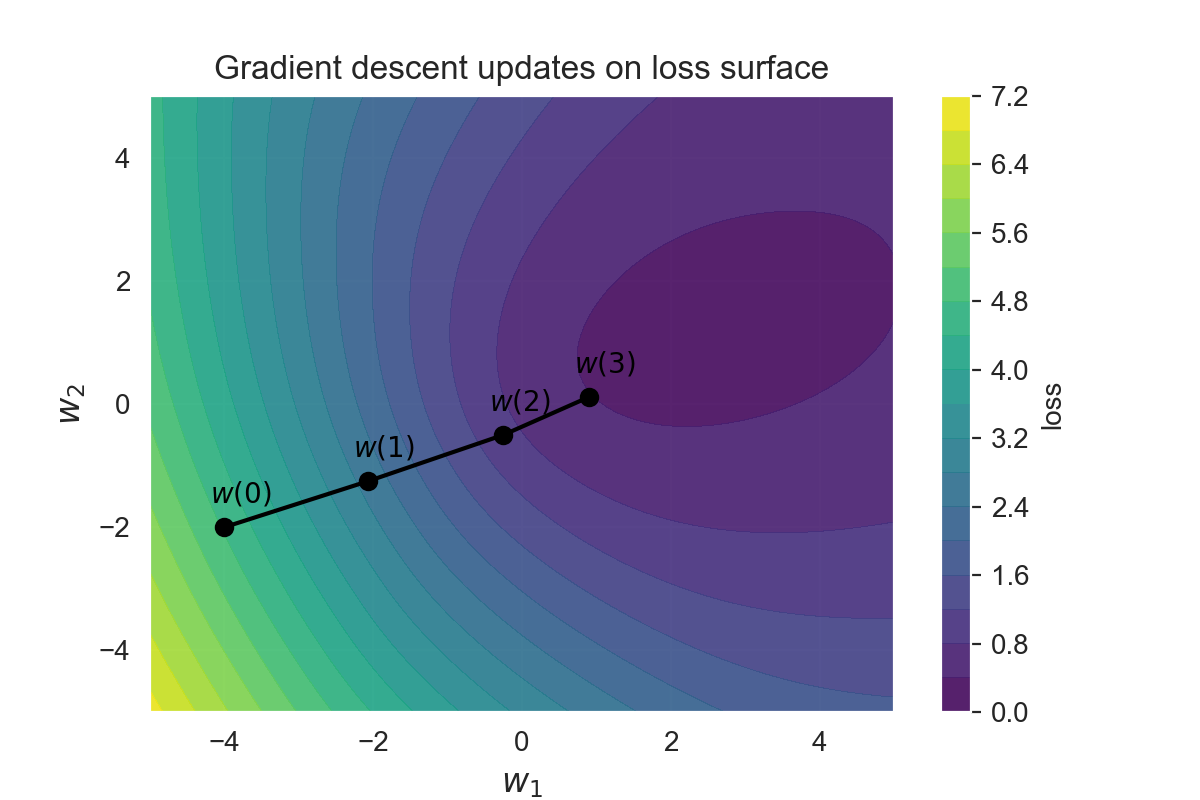## Visualization of the trained classifier ¶

The resulting decision boundary of running gradient descent on the example inputs $X$ and targets $\mathbf{t}$ is shown in the figure below. The background color refers to the classification decision of the trained classifier. Note that since this decision plane is linear that not all examples can be classified correctly. Two blue circles will be misclassified as red star, and four red stars will be misclassified as blue circles.

Note that the decision boundary goes through the point $(0,0)$ since we don't have a bias parameter on the logistic output unit.

In :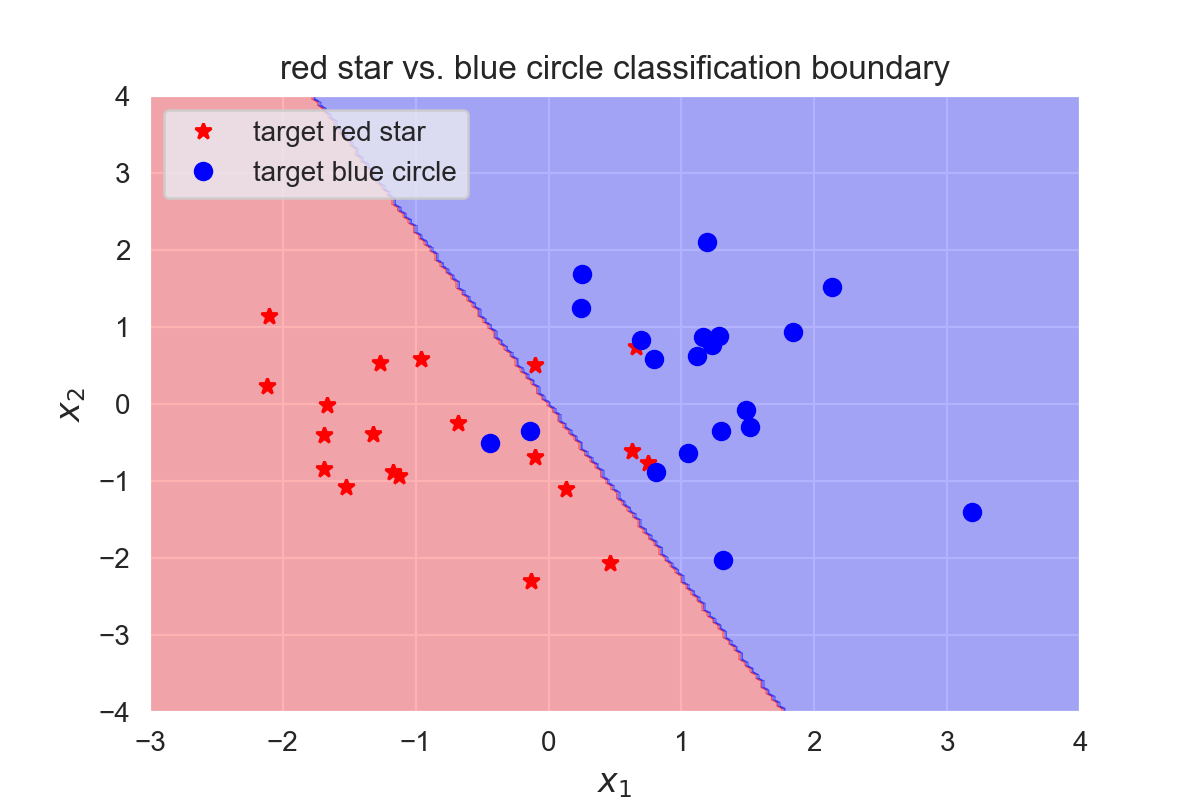In :
Python: 3.7.0
numpy: 1.15.2
matplotlib: 3.0.0
seaborn: 0.9.0


This was the second part of a 4-part tutorial on how to implement neural networks from scratch in Python:

This post at peterroelants.github.io is generated from an IPython notebook file. Link to the full IPython notebook file